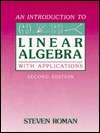##Introduction to Linear Algebra with Applications

This book provides a thorough introduction to Linear Algebra suitable for a first course. It can be used in a course with a theoretical flavor or one with emphasis on applications.

### Contents

• Systems of Linear Equations
• Systems of Equations and Matrices
• Gauss-Jordan Elimination
• Gaussian Elimination
• Applications: Systems of Equations in Everyday Life, Kirkhoff's Laws, Balancing Chemical Equations, Leontief's Model
• Matrices
• Matrix Arithmetic
• The properties of Matrix Arithmetic
• Row Multiplication and Matrix Multiplication; Finding the Inverse of a Matrix
• The System AX=B When A is Invertible
• The System AX=B When A is Not Invertible
• Applications: The Adjacency Matrix of a Graph, Markov Chains
• 1. Determinants
• Definition of the Determinant
• Expansion by Minors
• The Determinant and Elementary Row Operations
• The Determinant and Invertibility
• A Formula for A Inverse and Cramer's Rule
• 2. Two and Three Dimensional Euclidean Space
• The Definition of Euclidean Space, Vector Arithmetic
• The Properties of Vector Arithmetic, Collinearity, the Norm of a Vector
• The Dot Product, Projections
• Bases
• Orthonormal Bases
• The Cross Product
• Applications: Equations of Lines and Planes in Three-Dimensional Space
• 3. Vector Spaces
• The Definition of a Vector Space; Examples
• Subspaces
• The Linear Span of a Set of Vectors
• Bases and Linear Independence
• More on Bases and Linear Independence
• The Solution Space of a Homogeneous System
• The Row and Column Space of a Matrix
• Coordinate Matrices
• The Change of Basis Problem for Vectors
• 4. Linear Transformations
• Matrix Transformations
• Linear Transformations
• Some Simple Properties of Linear Transformations
• The Vector Space Lin(V,W); The Composition of Linear Transformations
• The Kernel and Range of a Linear Transformation
• Isomorphisms
• Linear Transformations from Rn to Rm
• The Matrix of a Linear Transformation
• The Change of Basis Problem for Linear Operators
• Applications: Plane Linear Transformations
• 5. Eigenvalues and Eigenvectors
• Eignevalues, Eignevectors and the Diagonalization of Matrices
• Computing the Eigenvalues and Eigenvectors of a Matrix
• More on the Diagonalization of Matrices
• The Diagonalizability of Linear Operators
• Applications: The Powers of a Diagonalizable Matrix and Recurrence Relations
• 6. Inner Product Spaces
• The Definition of an Inner Product Space
• Properties of the Inner Product
• Orthonormal Bases
• The Gram-Schmidt Process
• Orthogonal Diagonalizability
• 7. Applications
• Diffferential Equations
• Eigenfunctions and Orthogonal Sequences
• 8. Computational Methods
• Introduction; Floating Point Numbers and Roundoff Error
• Gaussian Elimination with parital Pivoting
• Conditioned Matrices
• Iterative Methods for Solving Systems of Equations
• Approximating Eigenvalues and Eigenvectors
• Appendix
• The Phrase "If and Only If"
• Functions

Second edition, 1988, ISBN 0-15-542736-9, 504 pp., Hardcover